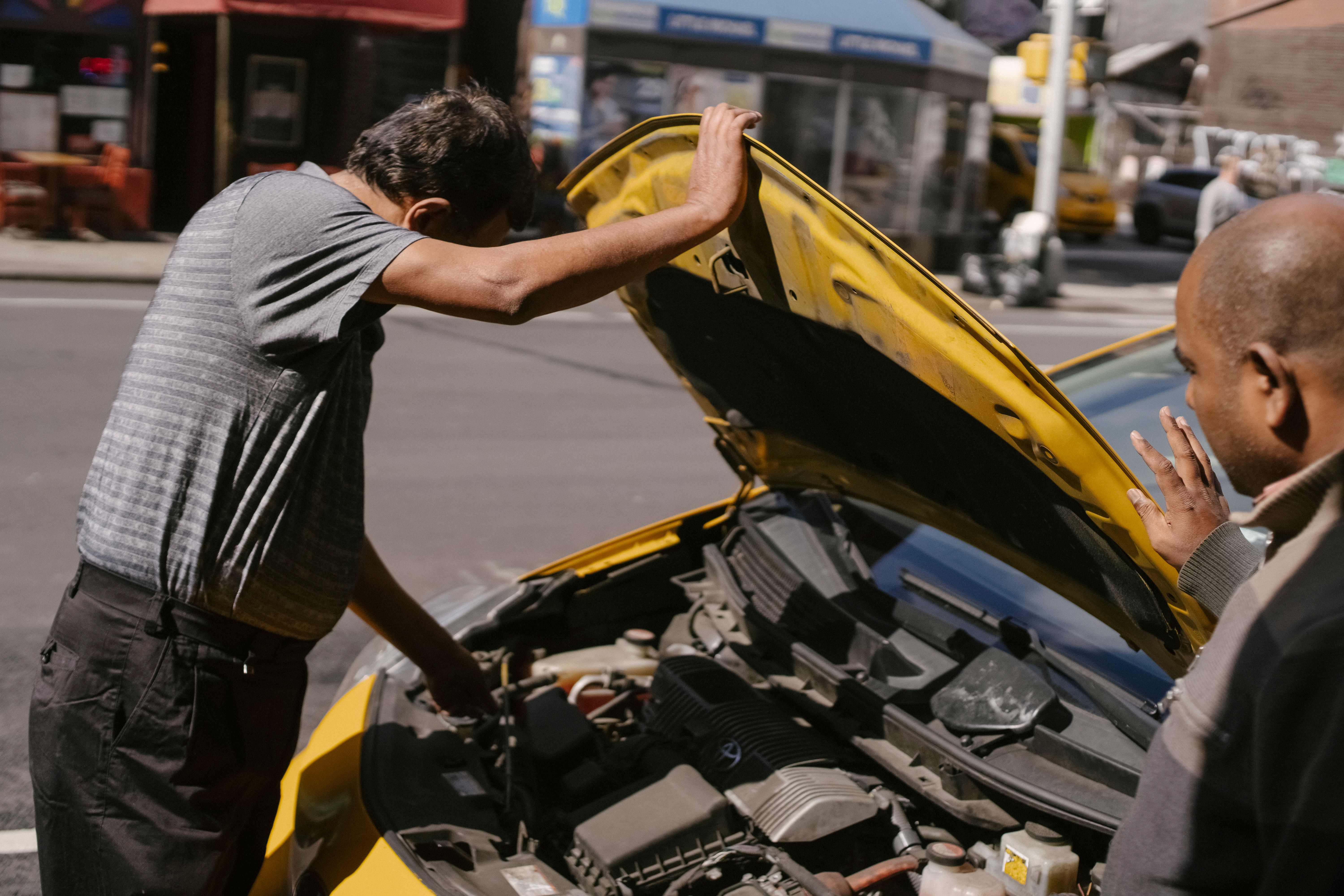# Inductive spiking, and how to fix it!

So in a few of my videos you might have
noticed that I put a diode in parallel with inductive loads like relays or motors. This video explains why that's important. First, make sure you've already seen my
tutorials about inductors and transistors so you know what's going on. So let's start out with a simple circuit
where I have a low side N channel MOSFET switching a purely resistive load of 20 ohms. We can pretend it's a small heater or something. And let's arbitrarily use a 6 volt power supply. We're going to be looking very
carefully at this particular node in the circuit, the transistor's "drain". If we use an external 7 volt source
we can switch the MOSFET on. When the MOSFET is on, there's almost
zero resistance across it, which means we have a direct connection to ground. So the voltage here is almost zero volts. This gives us a complete circuit. So current flows from the 6 volt supply,
through the resistor, through the transistor, and into the return current path.

Now let's apply 0 volts to the "gate"
of the MOSFET to turn it off. When the MOSFET is off, there's nearly
infinite resistance across it, so it's like it's not even in the circuit at all. Since no current can flow, there won't be
any voltage drop across the resistor. And since there's no voltage drop, 6 volts
minus zero means that we've got 6 volts right
here on the transistor's drain. And here's what that looks like on the oscilloscope. On the top I'm switching a MOSFET with a
7 volt square wave applied to the MOSFET's gate. And on the bottom you can see the
voltage at the transistor's drain. It's a 6 volt square wave. Very simple. Now let's get rid of that resistor
and make things a little more interesting. Here's the exact same circuit switching
an electric motor. uh… what the hell is happening here!? If we zoom out a bit we can see these
gigantic voltage spikes reaching 40V. So why is this happening? Well, the motor is an inductive load, so
let's model it as an inductor.

When the MOSFET is on, current flows
through the motor and everything is fine. Since the motor is running, now it has
some stored inductive energy. and let's represent that with this energy bar. Now when we switch the transistor off
look at what happens here. Remember the golden rule of inductors: The current in an inductor cannot
instantly change! So instantly after we turn off the transistor, there is still
current flowing through this inductor. In fact it's going to take a while for the
current to stop flowing. But there's nowhere for this current to flow! So what happens is that the voltage will
build up and up and up…

Until all of the stored inductive energy has been turned back into electrical energy. So we get this gigantic voltage spike on
the transistor's drain. Remember, the electron flow is actually
backwards compared to conventional current. So when current is flowing downwards here, all
the electrons are actually moving away. And with all the negative electrons leaving here we end up with a very positive voltage spike. And if the voltage spike is high enough
it runs the risk of destroying the transistor and other things attached to
this part of a circuit.I'm using an IRFZ44 which is
rated for up to 60V so I am lucky the inductive spiking wasn't enough to
kill it. But with a bigger motor driving a heavier load that could easily
have happened. Okay now how do we solve this problem? Well… put a diode in parallel with the
inductive load, but backwards. Now when the transistor switches off
there's actually a good path for current to flow. The inductive energy will get dumped out
of the motor through the diode and get returned to the power source.

And it will also recirculate back into the
motor until it dissipates. It's a simple addition to the circuit
and look at the difference it makes. The inductive spike is completely gone. Sometimes this diode is called a "reverse
bias diode", "antiparallel diode", "freewheeling diode", "catch diode",
or "flyback diode". And no matter what you call it, it's just
a diode that lets inductive energy take a return path somewhere safe. In this case, some of the energy is being
diverted back to the power supply's capacitors, recharging them just a little bit.

If the inductive load was much bigger, the returned energy might be too much
for the power supply to handle, in which case you'd need to be powering your
circuit from a battery that can be recharged. By the way, when you're doing this make
sure the diode is pointing in the right direction. If you have the diode this way around
current will continuously be flowing whenever the transistor is on and the
diode will blow up. Hooray! Another smelly failure! Now as for the type of diode, I used a
1N4007 and that will work in most situations under 1kHz. If you're switching things at higher
frequencies you should find a fast schottky diode like the classic
1N5819. Anyways that was just an example with
a motor but the exact same problem occurs with
any inductive load. This includes solenoids, the primary coil of a relay,
transformers in a flyback configuration, and a whole lot more. Alright thanks for watching. Now you
know how to save your circuits from inductive spiking..